Courses

# NCERT Solutions (Part- 3)- Factorisation Class 8 Notes | EduRev

## Mathematics (Maths) Class 8

Created by: Indu Gupta

## Class 8 : NCERT Solutions (Part- 3)- Factorisation Class 8 Notes | EduRev

The document NCERT Solutions (Part- 3)- Factorisation Class 8 Notes | EduRev is a part of the Class 8 Course Mathematics (Maths) Class 8.
All you need of Class 8 at this link: Class 8

Exercise 14.3

Question 1:
Carry out the following divisions: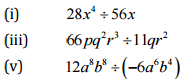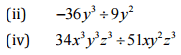(i)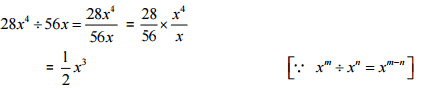(ii)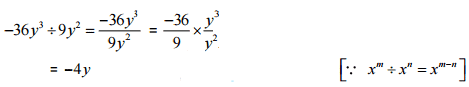(iii)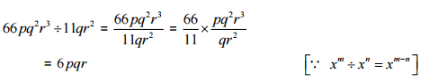(iv)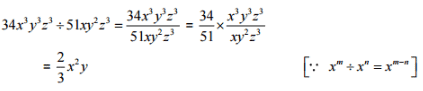(v)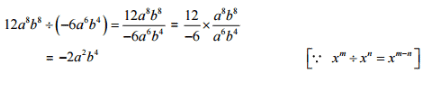Question 2:
Divide the given polynomial by the given monomial: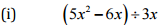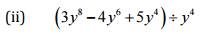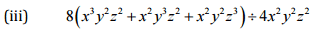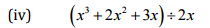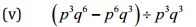(i)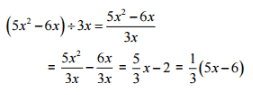(ii)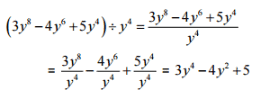(iii)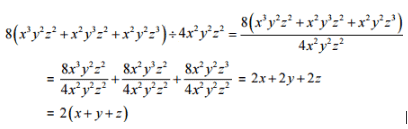(iv)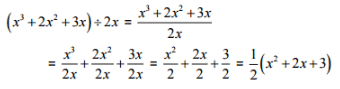(v)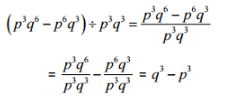Question 3:
Work out the following divisions: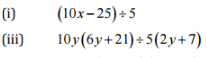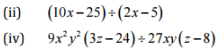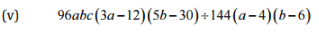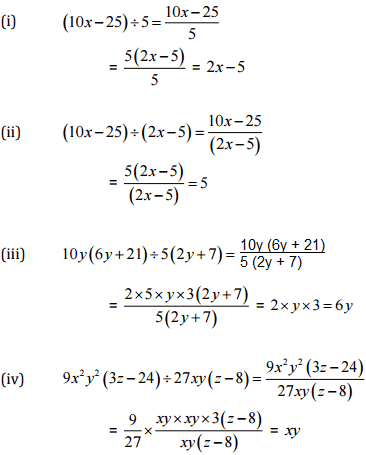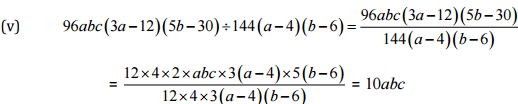Question 4:

Divide as directed: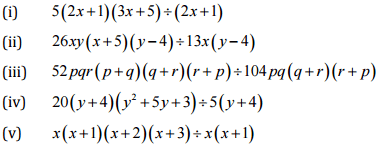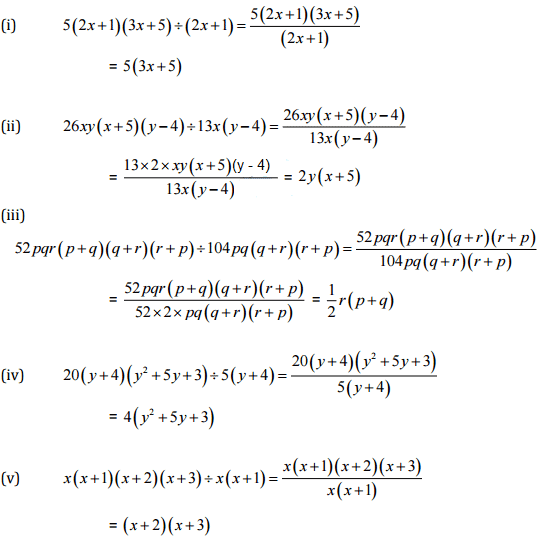Question 5:
Factorize the expressions and divide them as directed: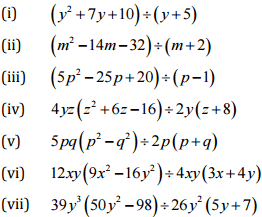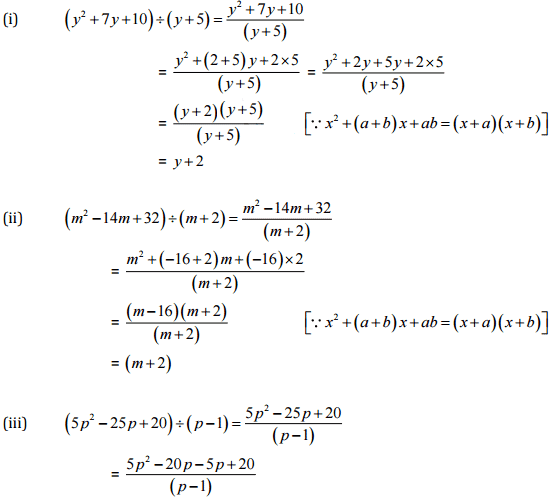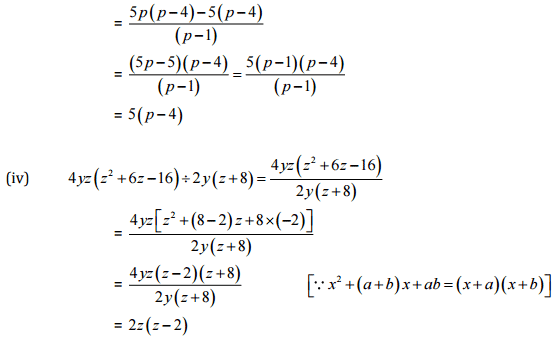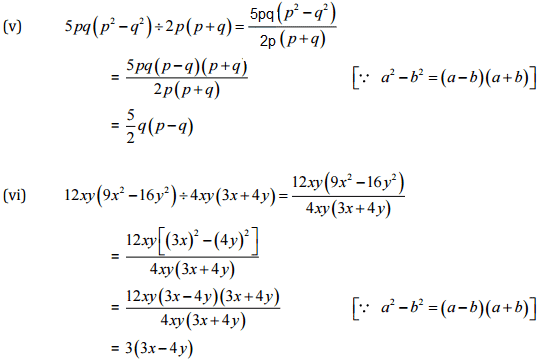,

,

,

,

,

,

,

,

,

,

,

,

,

,

,

,

,

,

,

,

,

;